Home > INT3 > Chapter 7 > Lesson 7.1.2 > Problem7-24

7-24.
1. Rewrite each equation as an equivalent equation using log base 10. You do not need to calculate a numerical answer. These are sometimes known as change of base problems. Homework Help ✎

1. log2(3) = x

2. log5(8) = x

3. log7(12) = x

4. loga(b) = x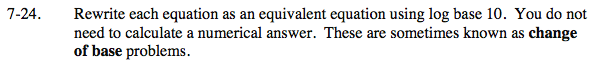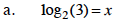Write the equation in exponential form.

2x = 3

Take the logarithm of base 10.

log10(2x) = log10(3)

Use the Power Property of Logarithms and solve for x.

xlog10(2) = log10(3)

$x= \frac{ \log_{10}(3) }{\log_{10}(2) }$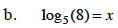Use the same process as in part (a).

$x= \frac{ \log_{10}(8) }{ \log_{10}(5)}$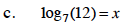Use the same process as in part (a).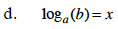Use the same process as in part (a).